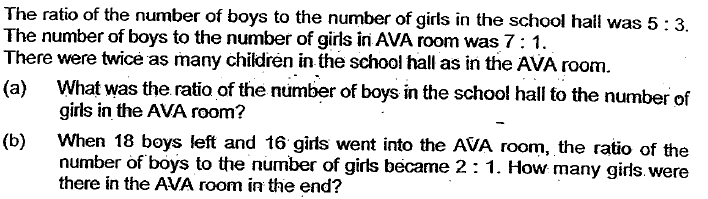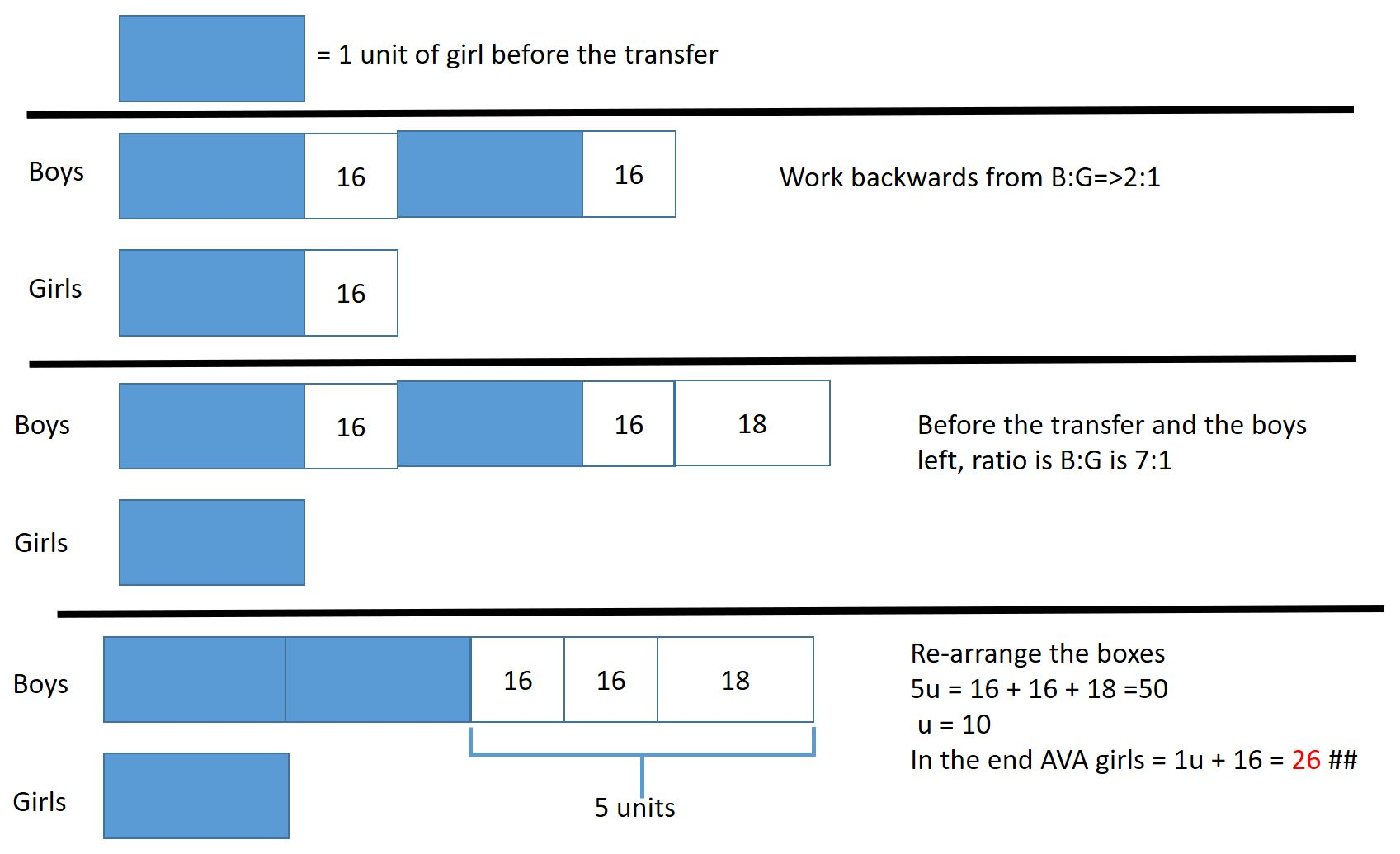QuestionHi, pls help to explain my mistake for Question part (a). Thanks.

my answer for (a) is B:G = 5:2

(a)
In the school hall,
boys : girls
5 : 3
10u : 3u

In the AVA room,
boys : girls
7u : 1u

ratio of the number of boys in the school hall to the number of girls in the AVA room is 10u : 1u = 10 : 1.

(b)
boys : 7u – 18
girls : 1u + 16

7u – 18 ——- (1u + 16) x 2 = 2u + 32
1u ——- (32 + 18)/(7 – 2) = 10
1u + 16 ——- 10 + 16 = 26

Ans : (a) 10 : 1; (b) 26 girls.

Thank you so much

You are most welcome.

0 Replies 0 Likes

This is similar to constant total concept, except that one total is twice of the other.

Strategy,
1. get the total of the ratio of the two group.
2. Work out mulitples of both the school hall and the AVA room, so that the total of the school hall is twice of AVA. In this case it’s easy, mulitple of 2 for school hall will work.

AVA room, B :G : Total => 7 : 1 : 8

School Hall, B : G : Total => 5 : 3 : 8 => 10 : 6 :16

Highlight in red now share common units (u).

a) School hall boys : AVA Girls => 10 : 1 ##

B) See attached picture.thank you.

0 Replies 0 Likes

Notice that the ratios given, 5+3=7+1. Then you can take ‘the number of children in school hall is twice as many as that in the AVA room’ literally. That is, B:G in the school hall is 10:6, while in the AVA room it is 7:1.

Thus for every girl in the AVA room, there are 10 boys in the school hall. Answer should be 10:1.

Your mistake is that ‘twice as many in A as in B’ means A=2B; there are more children in the school hall.

2 Replies 1 Like

HI Jyou, thanks.

0 Replies 0 Likes

Yup, poster twiced the wrong group of children. The group to be twiced is the school hall children.

0 Replies 1 Like